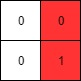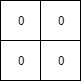1428. Leftmost Column with at Least a One

(This problem is an interactive problem.)

A binary matrix means that all elements are `0` or `1`. For each individual row of the matrix, this row is sorted in non-decreasing order.

Given a row-sorted binary matrix binaryMatrix, return leftmost column index(0-indexed) with at least a `1` in it. If such index doesn't exist, return `-1`.

You can't access the Binary Matrix directly.  You may only access the matrix using a `BinaryMatrix` interface:

• `BinaryMatrix.get(row, col)` returns the element of the matrix at index `(row, col)` (0-indexed).
• `BinaryMatrix.dimensions()` returns a list of 2 elements `[rows, cols]`, which means the matrix is `rows * cols`.

Submissions making more than `1000` calls to `BinaryMatrix.get` will be judged Wrong Answer.  Also, any solutions that attempt to circumvent the judge will result in disqualification.

For custom testing purposes you're given the binary matrix `mat` as input in the following four examples. You will not have access the binary matrix directly.

Example 1:```Input: mat = [[0,0],[1,1]]
Output: 0
```

Example 2:```Input: mat = [[0,0],[0,1]]
Output: 1
```

Example 3:```Input: mat = [[0,0],[0,0]]
Output: -1```

Example 4:```Input: mat = [[0,0,0,1],[0,0,1,1],[0,1,1,1]]
Output: 1
```

Constraints:

• `rows == mat.length`
• `cols == mat[i].length`
• `1 <= rows, cols <= 100`
• `mat[i][j]` is either `0` or `1`.
• `mat[i]` is sorted in a non-decreasing way.

1428. Leftmost Column with at Least a One
``````struct Solution;

struct BinaryMatrix {
data: Vec<Vec<i32>>,
}

impl BinaryMatrix {
fn new(data: Vec<Vec<i32>>) -> Self {
BinaryMatrix { data }
}
fn dimensions(&self) -> Vec<i32> {
vec![self.data.len() as i32, self.data.len() as i32]
}
fn get(&self, i: i32, j: i32) -> i32 {
self.data[i as usize][j as usize]
}
}

impl Solution {
fn left_most_column_with_one(matrix: &BinaryMatrix) -> i32 {
let d = matrix.dimensions();
let n = d;
let m = d;
let mut j = m;
while j > 0 {
let mut count = 0;
for i in 0..n {
while j > 0 && matrix.get(i, j - 1) == 1 {
count += 1;
j -= 1;
}
}
if count == 0 {
break;
}
}
if j == m {
-1
} else {
j as i32
}
}
}

#[test]
fn test() {
let data = vec_vec_i32![[0, 0], [1, 1]];
let matrix = BinaryMatrix::new(data);
let res = 0;
assert_eq!(Solution::left_most_column_with_one(&matrix), res);

let data = vec_vec_i32![[0, 0], [0, 1]];
let matrix = BinaryMatrix::new(data);
let res = 1;
assert_eq!(Solution::left_most_column_with_one(&matrix), res);

let data = vec_vec_i32![[0, 0], [0, 0]];
let matrix = BinaryMatrix::new(data);
let res = -1;
assert_eq!(Solution::left_most_column_with_one(&matrix), res);

let data = vec_vec_i32![[0, 0, 0, 1], [0, 0, 1, 1], [0, 1, 1, 1]];
let matrix = BinaryMatrix::new(data);
let res = 1;
assert_eq!(Solution::left_most_column_with_one(&matrix), res);
}
``````